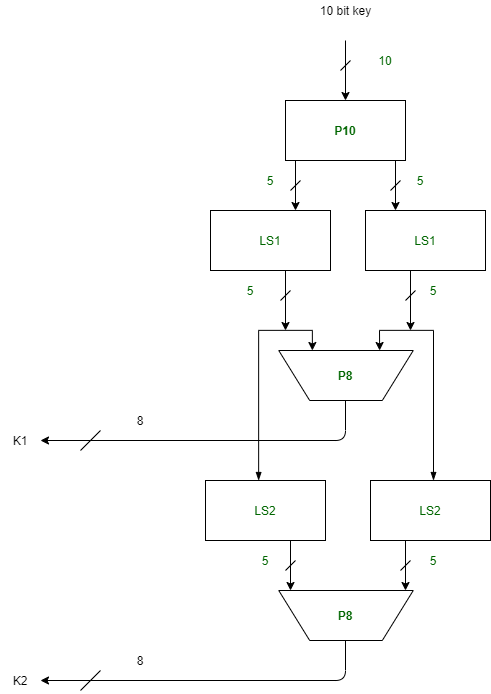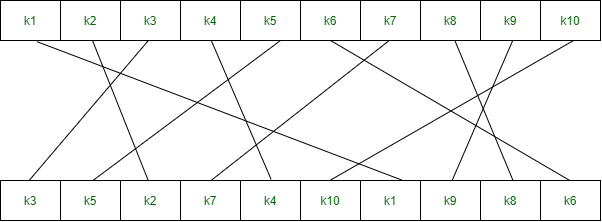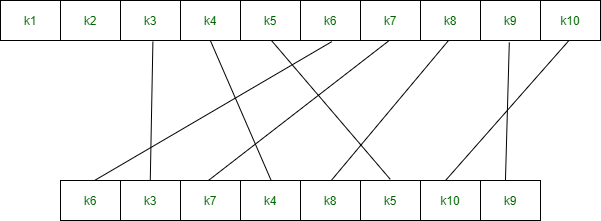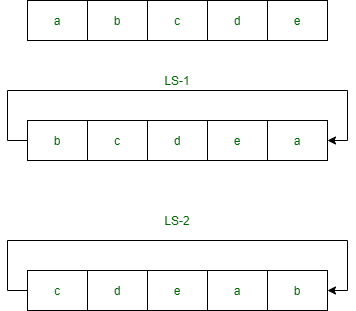GFG App
Open AppBrowser
Continue

# Simplified Data Encryption Standard Key Generation

Simplified Data Encryption Standard (S-DES) is a simple version of the DES Algorithm. It is similar to the DES algorithm but is a smaller algorithm and has fewer parameters than DES. It was made for educational purposes so that understanding DES would become simpler.  It is a block cipher that takes a block of plain text and converts it into ciphertext.  It takes a block of 8 bit.

It is a symmetric key cipher i.e. they use the same key for both encryption and decryption. In this article, we are going to demonstrate key generation for s-des encryption and decryption algorithm. We take a random 10-bit key and produce two 8-bit keys which will be used for encryption and decryption.

Key Generation Concept: In the key generation algorithm, we accept the 10-bit key and convert it into two 8 bit keys. This key is shared between both sender and receiver.In the key generation, we use three functions:

1. Permutation P102. Permutation P83. Left ShiftStep 1: We accepted a 10-bit key and permuted the bits by putting them in the P10 table.

```Key = 1 0 1 0 0 0 0 0 1 0
(k1, k2, k3, k4, k5, k6, k7, k8, k9, k10) = (1, 0, 1, 0, 0, 0, 0, 0, 1, 0)

P10 Permutation is: P10(k1, k2, k3, k4, k5, k6, k7, k8, k9, k10) = (k3, k5, k2, k7, k4, k10, k1, k9, k8, k6)
After P10, we get 1 0 0 0 0 0 1 1 0 0```

Step 2: We divide the key into 2 halves of 5-bit each.

`l=1 0 0 0 0, r=0 1 1 0 0`

Step 3: Now we apply one bit left-shift on each key.

`l = 0 0 0 0 1, r = 1 1 0 0 0 `

Step 4: Combine both keys after step 3 and permute the bits by putting them in the P8 table. The output of the given table is the first key K1.

```After LS-1 combined, we get 0 0 0 0 1 1 1 0 0 0
P8 permutation is: P8(k1, k2, k3, k4, k5, k6, k7, k8, k9, k10) = (k6, k3, k7, k4, k8, k5, k10, k9)
After P8, we get Key-1 : 1 0 1 0 0 1 0 0```

Step 5: The output obtained from step 3 i.e. 2 halves after one bit left shift should again undergo the process of two-bit left shift.

```Step 3 output - l = 0 0 0 0 1, r = 1 1 0 0 0
After two bit shift - l = 0 0 1 0 0, r = 0 0 0 1 1```

Step 6: Combine the 2 halves obtained from step 5 and permute them by putting them in the P8 table. The output of the given table is the second key K2.

```After LS-2 combined = 0 0 1 0 0 0 0 0 1 1
P8 permutation is: P8(k1, k2, k3, k4, k5, k6, k7, k8, k9, k10) = (k6, k3, k7, k4, k8, k5, k10, k9)
After P8, we get Key-2 : 0 1 0 0 0 0 1 1```

Final Output:

```Key-1 is: 1 0 1 0 0 1 0 0
Key-2 is: 0 1 0 0 0 0 1 1```
My Personal Notes arrow_drop_up